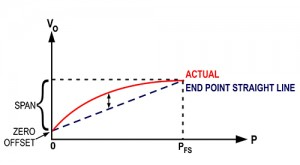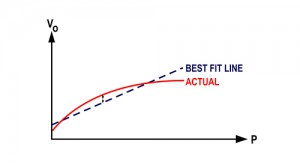### Scales

October 15, 2015

#### by Tom Lish

Which method provides better accuracy?  Non-linearity as the End Point Method is the relationship of the calibration curve to a specified straight line through its endpoints (i.e. Zero and Full Scale). Best Fit Straight Line (BFSL) method is the relationship of the calibration curve to a calculated straight line that minimizes the error but does not pass through the end points. BFSL method effectively cuts the end point value in half.

For a given non-linearity specification, a product specified with end point non-linearity will provide greater accuracy (i.e. lower uncertainty) than a product specified using BFSL method. The end point specification is more meaningful during re-calibration processes where the end points become Zero and Full Scale (FS) outputs.

### End Point MethodEnd point method measures non-linearity when a straight line is drawn connecting the end points from P0 (zero differential pressure) to PRS (full scale).  In this case, the end point accuracy is preserved when calibration adjustments are made to zero offset or span.

### Best Fit Straight Line MethodThe BFSL method of calculating non linearity fits a straight line through the actual curve in order to minimize the relative error between the actual and the straight line.  In this case, the end points of the curve have no meaningful relationship to the BFSL.  This results in a less accurate method of calculating non-linearity.

Setra's AccuSense™ Model ASM is a high performance industrial pressure transducer specifies non-linearity as the end-point method.. This is one of many reasons why industry leaders choose the ASM for high-tech applications, laboratories, aerospace applications, vacuum systems, dynamometers, engine test cells, and more.